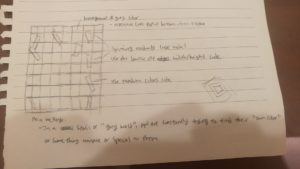# Week 2 Animation Assignment

For this week’s assignment, we were asked to incorporate both types of loops, specifically for() and while(), to showcase some various animations, loops, and conditionals.

During the process of making this, I had to go back and rewatch all of the previous class videos from the past two weeks, various Youtube videos online (Dan Shiffman’s are really helpful, will definitely watch more of his videos), and also some other coding tutorial websites and practices online in order to get a better understanding.

Originally, I wanted to make it  similar  to  this  sketch  of  mine,  but  I mainly  struggled  with  creating  the  floating  rectangles.  My   original  plan  was  to  use  the  loops  to  create  multiple  rectangles to float in  randomly  generated  directions  as  well  as  its  colors,  but I was  unable  to  do  it.  However,  I  will  make  sure  to  learn  it  in  my  spare  time.For this particular assignment, my main personal objective was to utilize both for and while loops in my code and to be able to generate random movements and animations as well as colors. Thought it may be extremely bright to look at due to the different colors changing randomly, I hope you guys like it. Another problem I encountered was when I wanted to use a void mousePressed() function where by clicking the mouse, all the colors would change back to grey or black colors as the compiler stated “unexpected token: void”. This can be found at the bottom of my code where a huge section was disabled with the //. I was unable to figure out why it had appeared this error.

```float angle = 100;
int linespace=72;
float y=0;
float x;
float xspeed=3;
float yspeed= 3;
float spacing=50;

void setup() {
size(1280, 1280);
rectMode(CENTER);
}

void draw() {
background(170);

stroke(150);
strokeWeight(2);

//Moving background lines
spacing=spacing+random(-1, 1);
//Vertical background lines
for (int x=0; x<width; x=x+20) {
line(x, 10, x, height);
x+=spacing;
}
//x=0;
//while(x<width){
//  line(x,10,x,height);
//  x+=spacing;
//}
y=0;
while (y<height) {
line(20, y, width, y);
y+=spacing;
}
//Failed attempt
for (int i=0; i<width; i += linespace) {
stroke(random(255), random(255), random(255));
line(i, i, i+5, i+height);
line(i, i+5, i+width, i+5);
//The retracting rectangle
x=0;
while (x<width) {
if (mouseX<1) {
x+=1;
} else {
x+=mouseX;
}
fill(random(255), random(255), random(255));
stroke(240);
rect(x, mouseY, 100, 100);
//line(i,i,i,i+height);
}
//Spining orange rectangles

pushMatrix();
//translate(width/2,height/2);
translate(mouseX, mouseY);
fill(random(255), random(255), random(255));
rotate(angle);
rect(mouseX, mouseY, 100, 100);
popMatrix();

fill(random(255), random(255), random(255));
rect(mouseX, mouseY, 100, 300);
angle+=.005;
}

//If mouse is pressed, make everthing stop changing colors
//  void mousePressed()){
//    for (int i=0; i<width; i += linespace) {
//      stroke(150);
//      line(i, i, i+5, i+height);
//      line(i, i+5, i+width, i+5);

//      x=0;
//      while (x<width) {
//        if (mouseX<1) {
//          x+=1;
//        } else {
//          x+=mouseX;
//        }
//        fill(150);
//        stroke(240);
//        rect(x, mouseY, 100, 100);

//        pushMatrix();
//        //translate(width/2,height/2);
//        translate(mouseX, mouseY);
//        fill(150);
//        rotate(angle);
//        rect(mouseX, mouseY, 100, 100);
//        popMatrix();

//        fill(150);
//        rect(mouseX, mouseY, 100, 300);
//        angle+=.005;

//      }
//    }
//  }
}```